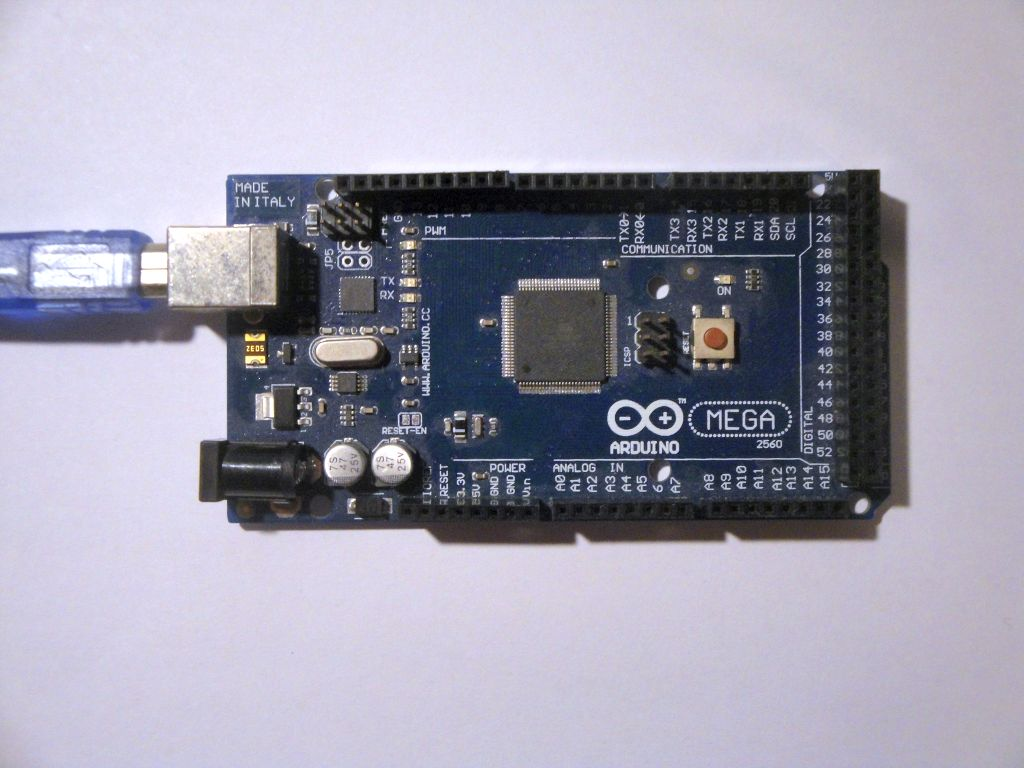# CTC timer in the ATmega2560 microcontroller

Zápisník experimentátora

Hierarchy:

The ATmega2560 microcontroller is part of the Arduino Mega 2560 board. It has more pins and its authors have added more timers. It includes two 8-bit and four 16-bit timers. In this article, we look at timers from a CTC mode viewpoint. We will use the online calculator to generate the programs.## Timers

The first three timers are identical to ATmega328P.

• Timer0 - 8-bit.
• Timer1 - 16-bit.
• Timer2 - 8-bit.

• Timer3 - 16-bit.
• Timer4 - 16-bit.
• Timer5 - 16-bit.

## Examples

For each timer I wrote a sample program that flashes with an internal LED at 1 Hz. In the examples, you will see that the 8-bit timer has to be set to a higher frequency and then you need to add an internal divider to get the desired frequency. With a 16-bit timer, this is not necessary and the desired frequency can be set directly.

### Example Timer0 - 8-bit

``````#define ledPin 13
volatile int divider=0;

void setupTimer0() {
noInterrupts();
// Clear registers
TCCR0A = 0;
TCCR0B = 0;
TCNT0 = 0;

// 100.16025641025641 Hz (16000000/((155+1)*1024))
OCR0A = 155;
// CTC
TCCR0A |= (1 << WGM01);
// Prescaler 1024
TCCR0B |= (1 << CS02) | (1 << CS00);
// Output Compare Match A Interrupt Enable
TIMSK0 |= (1 << OCIE0A);
interrupts();
}

void setup() {
pinMode(ledPin, OUTPUT);
setupTimer0();
}

void loop() {
}

ISR(TIMER0_COMPA_vect) {
if(divider==0)
divider++;
divider%=100;
}``````

### Example Timer1 - 16-bit

``````#define ledPin 13

void setupTimer1() {
noInterrupts();
// Clear registers
TCCR1A = 0;
TCCR1B = 0;
TCNT1 = 0;

// 1 Hz (16000000/((15624+1)*1024))
OCR1A = 15624;
// CTC
TCCR1B |= (1 << WGM12);
// Prescaler 1024
TCCR1B |= (1 << CS12) | (1 << CS10);
// Output Compare Match A Interrupt Enable
TIMSK1 |= (1 << OCIE1A);
interrupts();
}

void setup() {
pinMode(ledPin, OUTPUT);
setupTimer1();
}

void loop() {
}

ISR(TIMER1_COMPA_vect) {